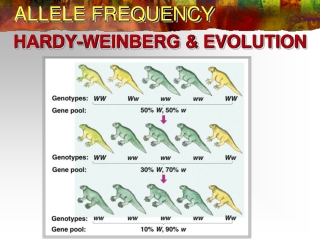DownloadDownload PresentationALLELE FREQUENCY

# ALLELE FREQUENCY

Télécharger la présentation## ALLELE FREQUENCY

- - - - - - - - - - - - - - - - - - - - - - - - - - - E N D - - - - - - - - - - - - - - - - - - - - - - - - - - -
##### Presentation Transcript

1. ALLELE FREQUENCY HARDY-WEINBERG & EVOLUTION

2. HARDY - WEINBERG • A population that is not changing genetically is said to be at Hardy–Weinberg equilibrium • The assumptions that underlie the Hardy–Weinberg equilibrium are • population is large • mating is random • There is no migration (no immigration or emigration) • There is no mutation of the alleles • natural selection is not acting on the population. (all genotypes have an equal chance of surviving and reproducing)

3. HARDY-WEINBERG & EVOLUTION • Biologists can determine whether an agent of evolution is acting on a population by comparing the population’s genotype frequencies with Hardy–Weinberg equilibrium frequencies. • If there is no change in frequencies, there is no evolution • Conversely, if there have been changes in the frequencies, then evolution has occurred. • Evolution is change of allelic frequencies

4. HARDY – WEINBERG formula • Two equations given on the formula sheet p + q = 1 • A + a = 1, where A and a equal gene percentages • All dominant alleles + all recessive alleles = all of the alleles for a particular gene in a population • Allele frequencies p2 + 2pq + q2 = 1 • AA + 2Aa + aa = 1 • For a particular gene, all homozygous dominant individuals + all heterozygous individuals + all homozygous recess individuals = all of the individuals in the population • Genotype frequencies

5. HARDY-WEINBERG PROBLEM • Given: In a population of 100 individuals (200 alleles), sixteen exhibit a recessive trait. • Problem: • Find the allele frequencies for A and a. • Find the genotypic frequencies of AA, Aa, and aa. • Allele frequency: • p + q = 1 or A + a = 1 • 16% = aa & 84% = AA +Aa • aa = qq or q2 = .16 or q = .4 • 1 - q = p 1 - .4 = .6 or A = .6 and a = .4

6. HARDY - WEINBERG PROBLEM • Phenotypic frequencies • If: p = .6 and q = .4, then • p2 = (.6)(.6) = .36 • q2 = (.4)(.4) = .16 • 2pq = 2(.6)(.4) = .48 • Therefore, in the population: • Homozygous dominant = 36/100 or 36% • Heterozygous dominant = 48/100 or 48% • Recessive = 16/100 or 16%

7. ANOTHER PROBLEM • Fraggles are mythical, mouselike creatures that live beneath flower gardens. • Of the 100 fraggles in a population, 91 have green hair(F) and 9 have grey hair(f). • Assuming genetic equilibrium: • What are the gene frequencies of F and f? • What are the genotypic frequencies?

8. ANSWERS TO PROBLEM • Gene frequencies: • F = 0.7 and f = 0.3 • Genotypic frequencies • FF = 49% or 0.49 • Ff = 42% or 0.42 • f f = 9% or .09